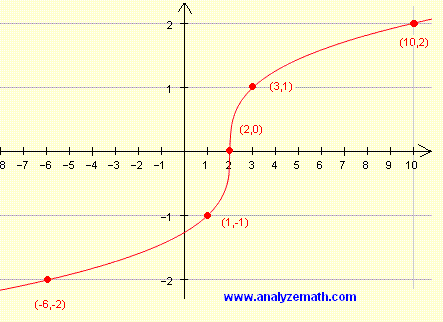# Graphing Cube Root Functions

A tutorial on graphing and sketching cube root functions is presented. The graph, domain, range of these functions and other properties are discussed.

## Graph, Domain and Range of the Basic Cube Root Function: f(x) = ∛x

The domain of function f defined by f(x) = ∛x is the set of all real numbers.
The range of f is the set of all real numbers.

### Example 1

Graph
f( x ) = ∛x
and find the range of f.
Solution to Example 1
Because the domain of f is the set of all real numbers, we might construct a table of values as follows:
 x -8 1 0 1 8 f(x) = ∛x -2 1 0 1 2

The values of x were selected so that the cube root of these values are whole numbers which make it easy to plot the points shown in the table.The range of f is given by the interval (-∞ , +∞).

## Graph the More General Cube Root Function: f(x) = ∛x

### Example 2

Graph
f( x ) = ∛ (x - 2)

and find the range of f.
Solution to Example 2
The domain of the cube root function given above is the set of all real numbers.
It easy to calculate ∛ (x - 2)if you select values of (x - 2) as -8, -1, 0, 1 and 8 to construct a table of values then find x in order to graph f.
 x - 2 -8 -1 0 1 8 f(x) = ∛ (x - 2) -2 -1 0 1 2 x -6 1 2 3 10

The last two rows in the table of data are used to graph f.The range of f is the set of all real numbers.
Note also that the graph of f(x) = ∛ (x - 2) is that of f(x) = ∛ ( x ) shifted 2 units to the right.

### Example 3

Graph
f( x ) = - ∛(x + 1)
and find the range of f.
Solution to Example 3
The domain of the function given above is the set of all real numbers
We now select values of (x + 1) as -8, -1, 0, 1 and 8 to construct a table of values then find x in order to graph f .

 x + 1 -8 -1 0 1 8 f(x) = - ∛ (x + 1) 2 1 0 - 1 - 2 x -9 -2 -1 0 7The range of f is given by the interval (-∞ , +∞).

### Example 4

Graph
f( x ) = - 2 ∛ (x - 2) + 2
and find the range of f.

Solution to Example 4
The domain of function f is the set of all real values.
We now select values of (x - 2) as -8, -1, 0, 1 and 8 to construct a table of values.

 x - 2 -8 -1 0 1 8 f(x) = - 2 ∛ (x - 2) + 2 6 4 2 0 - 2 x -6 1 2 3 10The range of f is the set of all real numbers.

## More References and Links to Graphing

Graphing Functions